# 10 PRINT mazes with ggplot2

Want to share your content on R-bloggers? click here if you have a blog, or here if you don't.

There is a celebrated Commodore 64 program that randomly prints outs `/` and `\`
characters and fills the screen with neat-looking maze designs. It is just one
line of code, but there is a whole book written about
it.

``````10 PRINT CHR\$(205.5+RND(1)); : GOTO 10
``````Screenshots of the 10 PRINT program in action. Images taken from the 10 PRINT book.

The basic idea, from my reading of the code, is that `CHR\$(205)` is `\`,
`CHR\$(206)` is `/`, and the program randomly selects between the two by adding a
random number to 205.5. Endlessly looping over this command fills the screen
with that pleasing maze pattern.

In R, we could replicate this functionality with by randomly sampling the
slashes:

``````sample_n_slashes <- function(n) {
sample(c("/", "\\"), size = n, replace = TRUE)
}

withr::with_options(
list(width = 40),
cat(sample_n_slashes(800), sep = "", fill = TRUE)
)
#> //////\/\\/\/\/\/\\//\\\\//\//\/\//\///\
#> \\\/\//\\/\/////////////\\/\//\\\/\//\\\
#> /\\/\/\//\/\/\\/\\/\/\//\\\\//\/\\\/\///
#> \\//\/\\\/\///\\/\/\/////\//\///\/\/\//\
#> /\/\//\///\//\//\//\/\//\\/\\\\\/\\\//\/
#> //\\\\\//////\//\//\/\//\\////\/\\\/\/\/
#> \\////\/\\/\////\//////\\/\\/////\/////\
#> /\/\/\/\/\\///\/\\\\/\/\\//\/\\/\\\\\\//
#> //\\\/\///\///\/\\\//\//\\\\//\\/\\/\/\/
#> /\\/\//\\//\/\\\\\/\\\/\\\/\/\/\/\////\/
#> /\//\\//\////\\\///\//\/\\\/\\///\//\\\\
#> /\//\\////\/\\\//\\\//\/\///\\\\\/\/\\//
#> \\////\\/\\\\\\///\///\//\\\/\\\\//\////
#> \\\///\/\//\//\/\//\/\/\\\/\/\\///\/////
#> \\/\\//\\\\\\///\\\\/\\/\\//\//\\\/\\/\/
#> ////\\\////\/\\\\\/////\/\\\////\\///\\\
#> \//\\\//\///\/\\\\//\\\////\\//\\/\/\//\
#> \/\//\\//\\///\\\\\\//\///\/\\\\\\\\/\\/
#> ///\/\//\\/\/\\\\\\\\/\///\//\\///\\//\\
#> /////\\///\/\\/\/\\//\\//\/\\/\//\//\\\\
``````

where `withr::with_options()` lets us temporarily change the print width and
`cat()` concatenates the slashes and prints out the characters as text.

We can also make this much prettier by drawing the patterns using ggplot2.

## Drawing line segments with ggplot2

Instead of writing out slashes, we will draw a grid of diagonal line segments,
some of which will be flipped at random. To draw a segment, we need a starting
xy coordinate and an ending xy coordinate. `geom_segment()` will
connect the two coordinates with a line. Here’s a small example where we draw
four “slashes”.

``````library(ggplot2)
library(dplyr)

data <- tibble::tribble(
~row, ~col, ~x_start, ~x_end, ~y_start, ~y_end,
1,    1,        0,      1,        0,      1,
1,    2,        1,      2,        1,      0, # flipped
2,    1,        0,      1,        1,      2,
2,    2,        1,      2,        1,      2)

ggplot(data) +
aes(x = x_start, xend = x_end, y = y_start, yend = y_end) +
geom_segment()
``````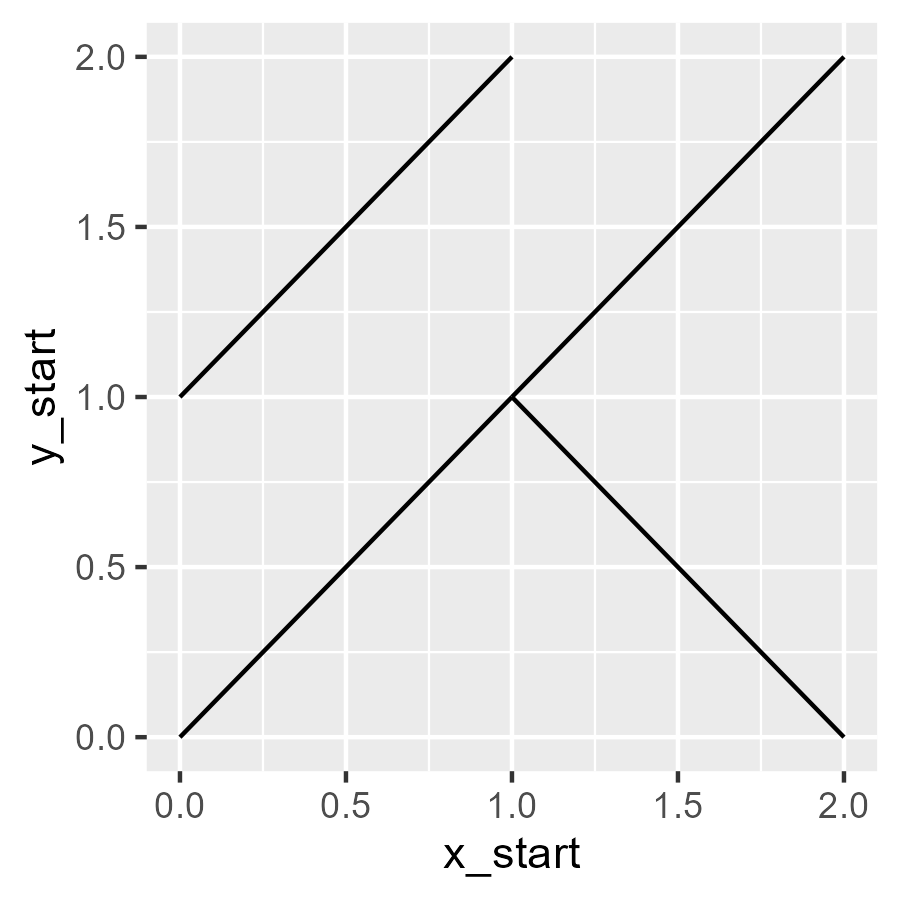The programming task now is to make giant grid of these slashes. Let’s start
with an observation: To draw two slashes, we needed three x
values: 0, 1, 2. The first two served as segment starts and the last two
as segment ends. The same idea applies to the y values. We can generate a
bunch of starts and ends by taking a sequence of steps and removing the first
and last elements.

``````# We want a 20 by 20 grid
rows <- 20
cols <- 20

x_points <- seq(0, 1, length.out = cols + 1)
x_ends <- tail(x_points, -1)

y_points <- seq(0, 1, length.out = rows + 1)
y_ends <- tail(y_points, -1)
``````

Each `x_starts``x_ends` pair is a column in the grid, and each
`y_starts``y_ends` is a row in the grid. To make a slash at each
row–column combination, we have to map out all the combinations of the rows
and columns. We can do this with `crossing()` which creates all crossed
combinations of values. (If it helps, you might think of crossed like crossed
experiments
or the
Cartesian cross product of
sets.)

``````grid <- tidyr::crossing(
# columns
data_frame(x_start = x_starts, x_end = x_ends),
# rows
data_frame(y_start = y_starts, y_end = y_ends)) %>%
# So values move left to right, bottom to top
arrange(y_start, y_end)

# 400 rows because 20 rows x 20 columns
grid
#> # A tibble: 400 x 4
#>    x_start x_end y_start y_end
#>
#>  1    0     0.05       0  0.05
#>  2    0.05  0.1        0  0.05
#>  3    0.1   0.15       0  0.05
#>  4    0.15  0.2        0  0.05
#>  5    0.2   0.25       0  0.05
#>  6    0.25  0.3        0  0.05
#>  7    0.3   0.35       0  0.05
#>  8    0.35  0.4        0  0.05
#>  9    0.4   0.45       0  0.05
#> 10    0.45  0.5        0  0.05
#> # ... with 390 more rows
``````

We can confirm that the segments in the grid fill out a plot. (I
randomly color the line segments to make individual ones visible.)

``````ggplot(grid) +
aes(
x = x_start, y = y_start,
xend = x_end, yend = y_end,
color = runif(400)) +
geom_segment(size = 1) +
guides(color = FALSE)
``````Finally, we need to flip slashes at random. A segment becomes flipped if the
`y_start` and `y_end` are swapped. In the code below, we flip the slash in each
row if a randomly drawn number between 0 and 1 is less than .5. For style, we
also use `theme_void()` to strip away the plotting theme, leaving us with just
the maze design.

``````p_flip <- .5

grid <- grid %>%
arrange(y_start, y_end) %>%
mutate(
p_flip = p_flip,
flip = runif(length(y_end)) <= p_flip,
y_temp1 = y_start,
y_temp2 = y_end,
y_start = ifelse(flip, y_temp2, y_temp1),
y_end = ifelse(flip, y_temp1, y_temp2)) %>%
select(x_start:y_end, p_flip)

ggplot(grid) +
aes(x = x_start, y = y_start, xend = x_end, yend = y_end) +
geom_segment(size = 1, color = "grey20")

last_plot() + theme_void()
``````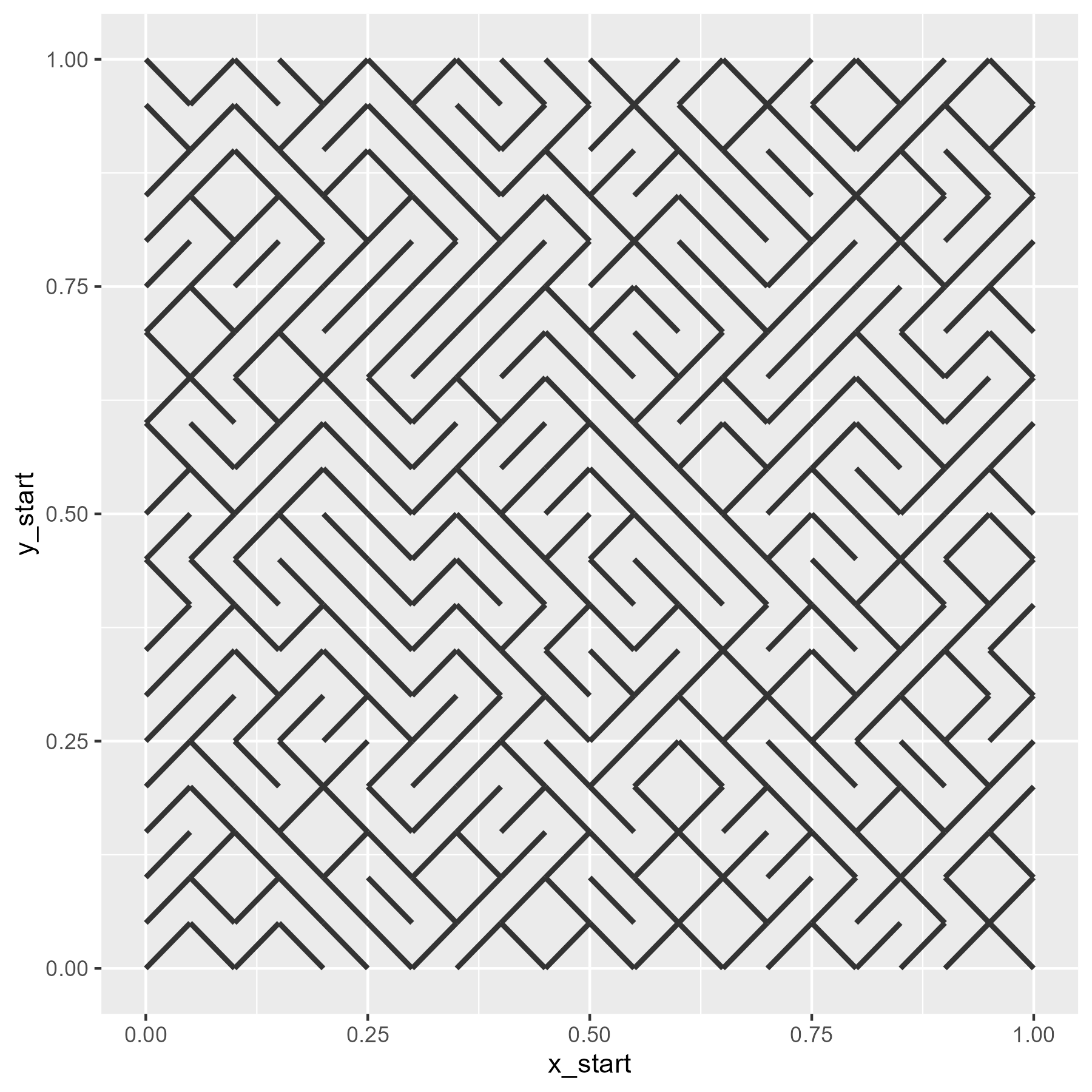Now, we wrap all these steps together into a pair of functions.

``````make_10_print_data <- function(rows = 20, cols = 20, p_flip = .5) {
x_points <- seq(0, 1, length.out = cols + 1)
x_ends <- tail(x_points, -1)

y_points <- seq(0, 1, length.out = rows + 1)
y_ends <- tail(y_points, -1)

grid <- tidyr::crossing(
data.frame(x_start = x_starts, x_end = x_ends),
data.frame(y_start = y_starts, y_end = y_ends))

grid %>%
arrange(y_start, y_end) %>%
mutate(
p_flip = p_flip,
flip = runif(length(y_end)) <= p_flip,
y_temp1 = y_start,
y_temp2 = y_end,
y_start = ifelse(flip, y_temp2, y_temp1),
y_end = ifelse(flip, y_temp1, y_temp2)) %>%
select(x_start:y_end, p_flip)
}

draw_10_print <- function(rows = 20, cols = 20, p_flip = .5) {
grid <- make_10_print_data(rows = rows, cols = cols, p_flip = p_flip)

ggplot(grid) +
aes(x = x_start, y = y_start, xend = x_end, yend = y_end) +
geom_segment(size = 1, color = "grey20")
}
``````

## Now the fun part: custom flipping probabilities

We can vary the probability of flipping the slashes. For example, we can use the
density of a normal distribution to make flipping more likely for central values
and less likely for more extreme values.

``````xs <- seq(0, 1, length.out = 40)
p_flip <- dnorm(seq(-4, 4, length.out = 40))

ggplot(data.frame(x = xs, y = p_flip)) +
aes(x, y) +
geom_line() +
labs(
x = "x position",
y = "p(flipping)",
title = "normal density")

# We repeat p_flip for each row of the grid
draw_10_print(rows = 40, cols = 40, p_flip = rep(p_flip, 40)) +
theme_void()
``````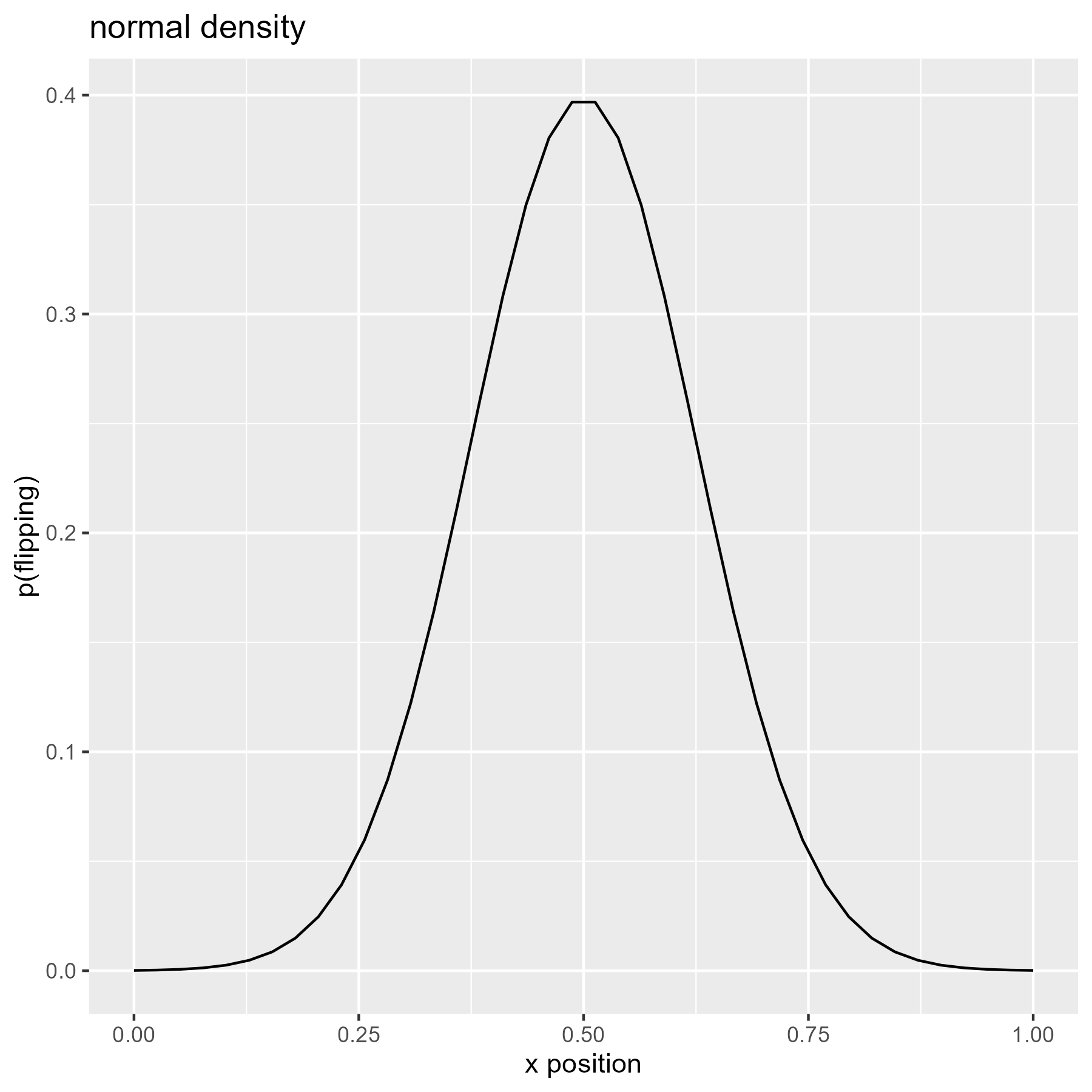We can use the cumulative density of the normal distribution so that
flipping becomes more likely as x increases.

``````xs <- seq(0, 1, length.out = 40)
p_flip <- pnorm(seq(-4, 4, length.out = 40))

ggplot(data.frame(x = xs, y = p_flip)) +
aes(x, y) +
geom_line() +
labs(
x = "x position",
y = "p(flipping)",
title = "cumulative normal")

draw_10_print(rows = 40, cols = 40, p_flip = rep(p_flip, 40)) +
theme_void()
``````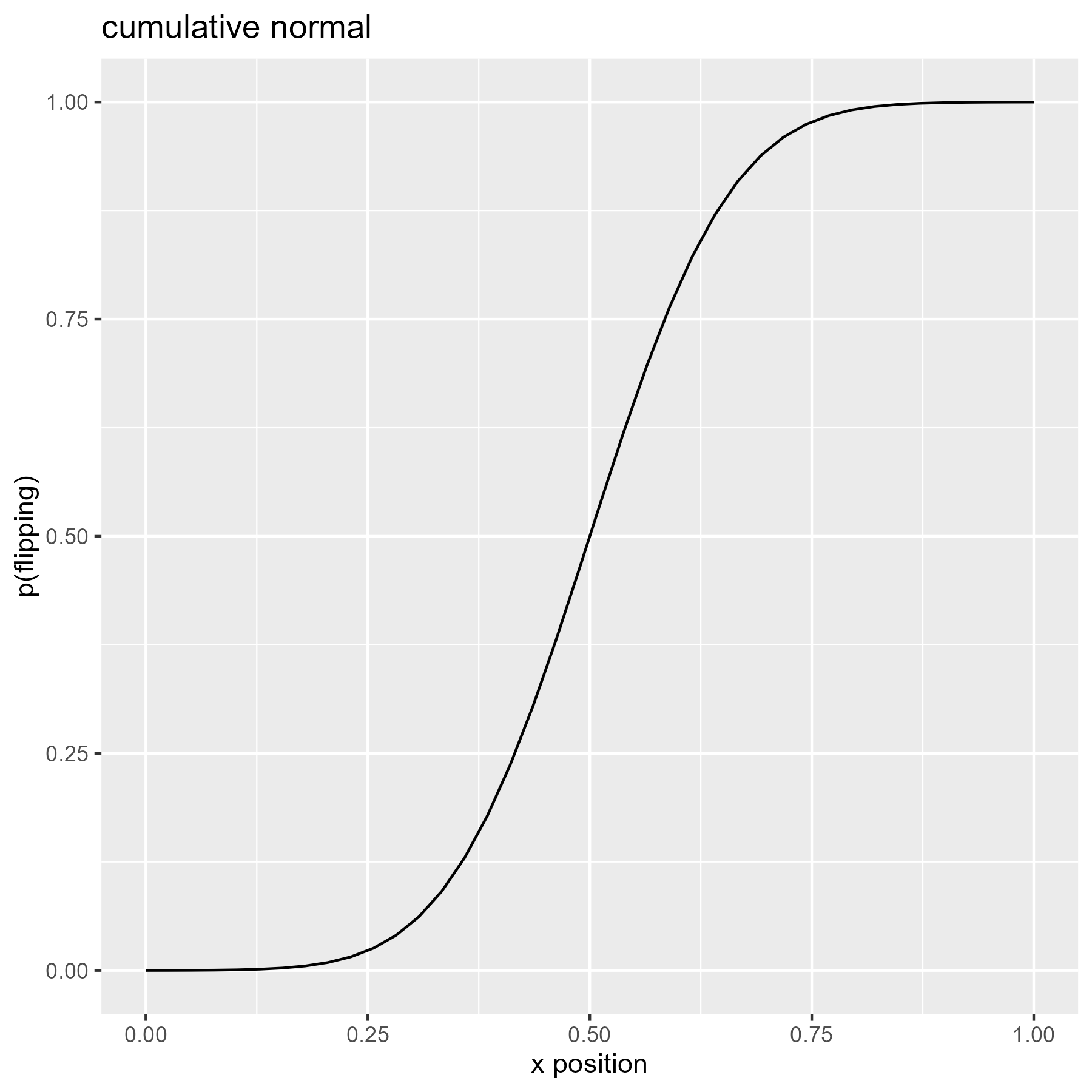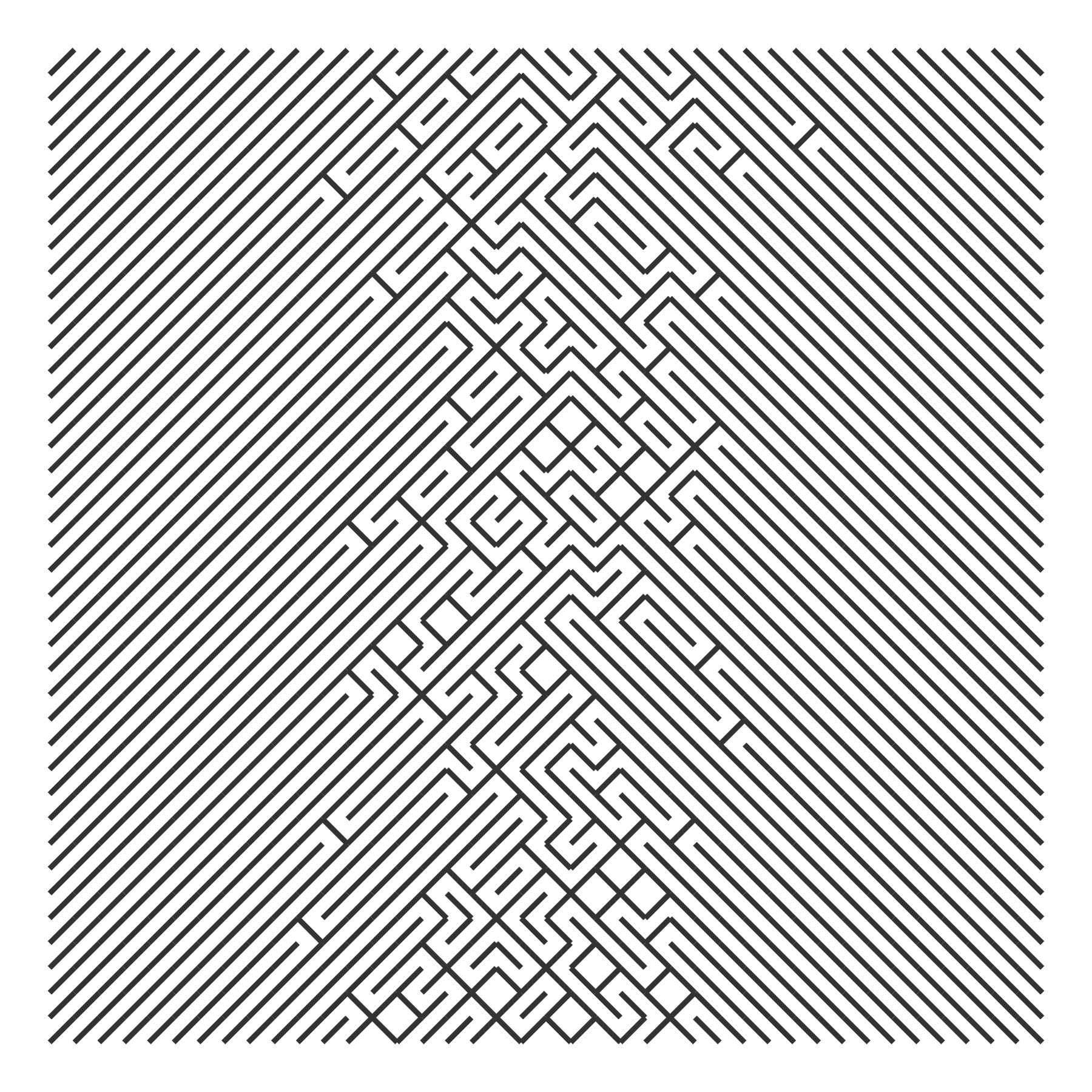The Cauchy distribution is said to have “thicker” tails than the normal
distribution, so here it shows more flips on the left and right extremes.

``````xs <- seq(0, 1, length.out = 40)
p_flip <- dcauchy(seq(-4, 4, length.out = 40))

ggplot(data.frame(x = xs, y = p_flip)) +
aes(x, y) +
geom_line() +
labs(
x = "x position",
y = "p(flipping)",
title = "Cauchy density")

draw_10_print(rows = 40, cols = 40, p_flip = rep(p_flip, 40)) +
theme_void()
``````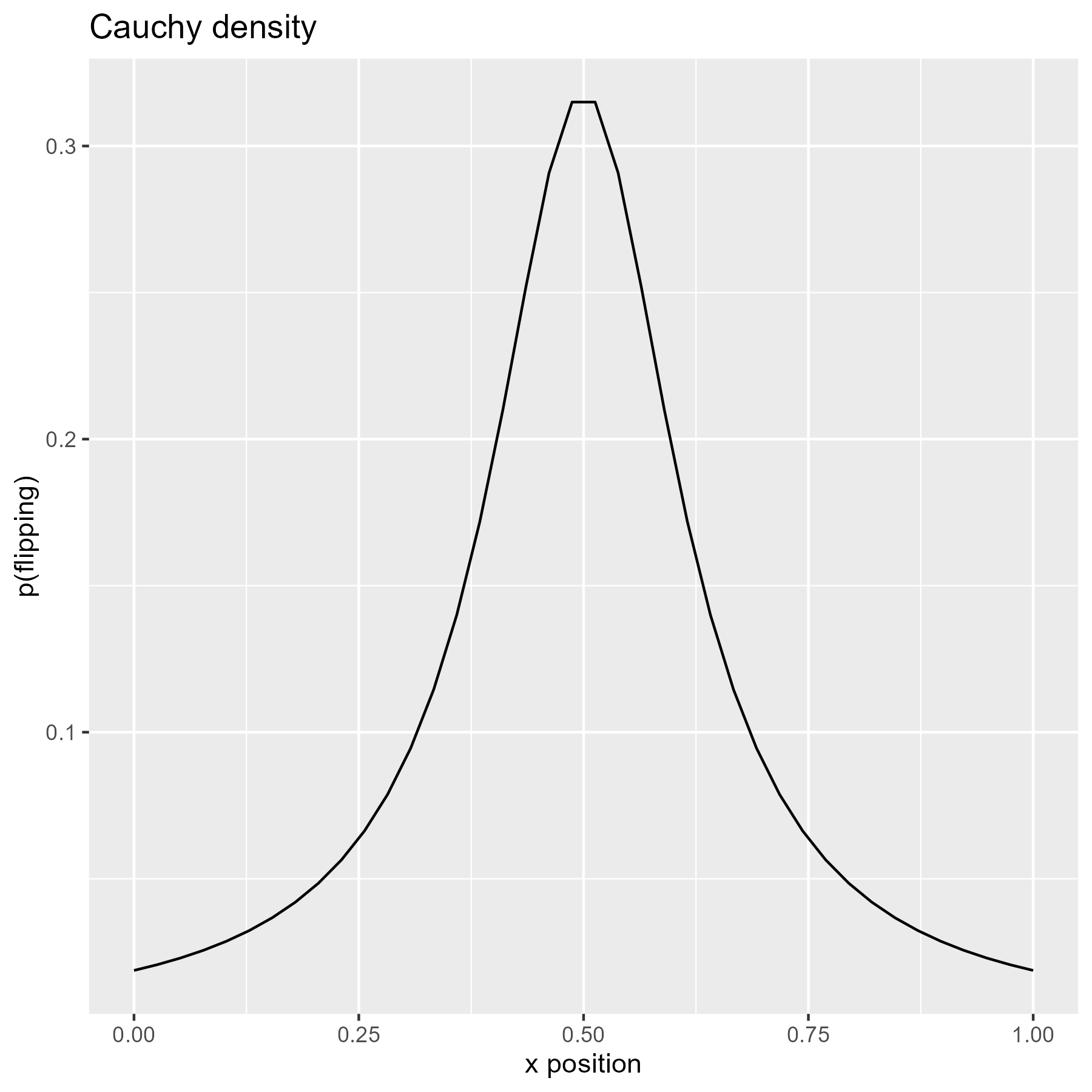The exponential distribution is a spike that quickly peters out. We can make a
probability “bowl” by splicing an exponential and a reversed exponential
together.

``````# Use flipped exponential densities as probabilities
p_flip <- c(dexp(seq(0, 4, length.out = 20)),
dexp(seq(4, 0, length.out = 20)))

ggplot(data.frame(x = xs, y = p_flip)) +
aes(x, y) +
geom_line() +
labs(
x = "x position",
y = "p(flipping)",
title = "exponential + flipped exponential")

draw_10_print(rows = 40, cols = 40, p = rep(p_flip, 40)) +
theme_void()
``````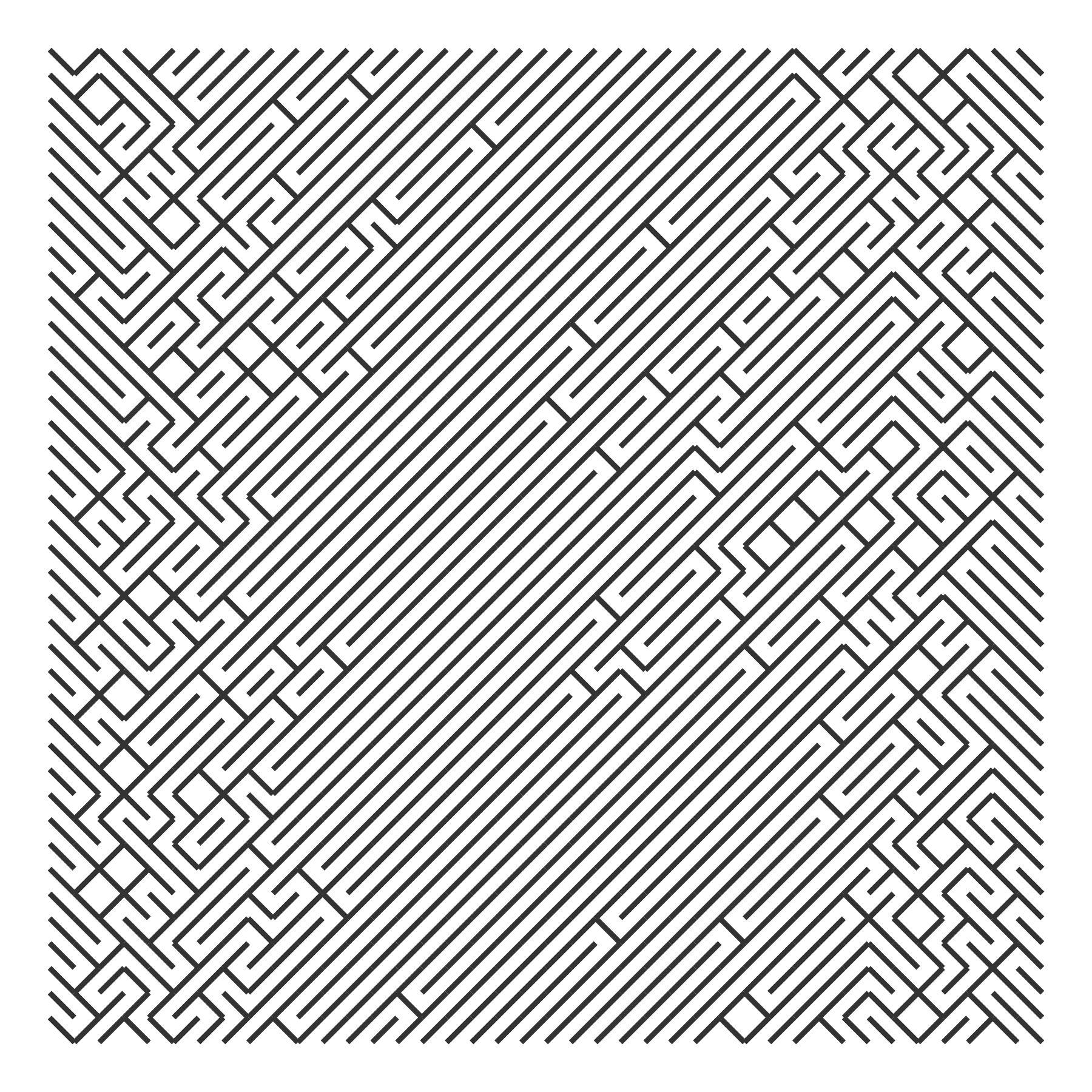We might have the probabilities increase by 10% from row to row. In the code
below, I use a simple loop to boost some random probability values by 10% from
row to row. This gives us nice streaks in the grid as a column starts to flip
for every row.

``````boost_probs <- function(p_flip, nrows, factor = 1.1) {
output <- p_flip
for (i in seq_len(nrows - 1)) {
p_flip <- p_flip * factor
output <- c(output, p_flip)
}
output
}

draw_10_print(cols = 40, rows = 40, p = boost_probs(runif(40), 40, 1.1)) +
theme_void()
``````The probabilities can be anything we like. Here I compute the frequency of
English alphabet letters as they appear in Pride and Prejudice and based the
flipping probability on those values.

``````char_counts <- janeaustenr::prideprejudice %>%
tolower() %>%
stringr::str_split("") %>%
unlist() %>%
table()

letter_counts <- char_counts[letters] %>% as.vector()
p_letter <- letter_counts / sum(letter_counts)

ggplot(data.frame(x = letters, y = p_letter)) +
aes(x, y, label = x) +
geom_text() +
labs(
x = NULL,
y = "p(letter)",
title = "letter frequencies in Pride and Prejudice")
````````````draw_10_print(cols = 26, rows = 80, p = rep(p_letter, 80)) +
theme_void()
``````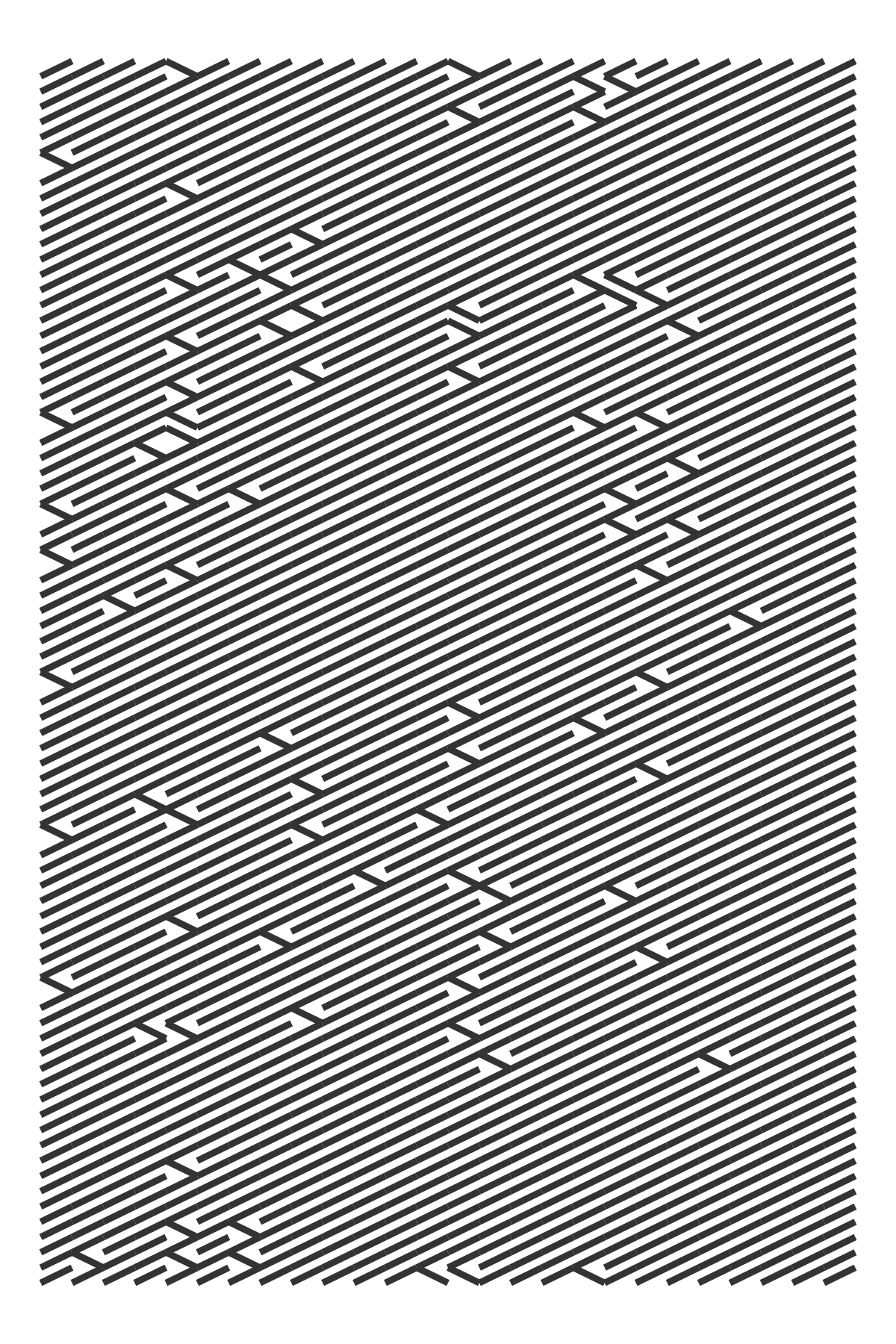R-bloggers.com offers daily e-mail updates about R news and tutorials about learning R and many other topics. Click here if you're looking to post or find an R/data-science job.
Want to share your content on R-bloggers? click here if you have a blog, or here if you don't.